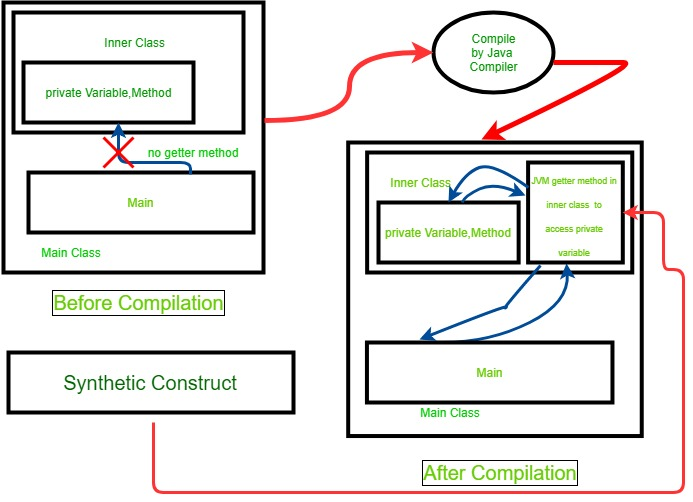GFG App
Open AppBrowser
Continue

# Method class isSynthetic() method in Java

java.lang.reflectMethod class help us to get information of a single method on a class or interface. This class also provides access to the methods of classes and invoke them at runtime.

isSynthetic() method of Method class: This function checks whether Method Object is a synthetic construct or not. If the method is a synthetic construct then the function returns true otherwise it will return false.

Synthetic Construct: Synthetic Construct are Class, Fields, and Methods that are created by the Java compiler for internal purposes.Syntax:

`public boolean isSynthetic()`

Return Value: This method returns true if and only if Method is a synthetic construct as specified by the JVM. Else it returns false.

Below programs illustrates isSynthetic() method of Method class:

Example 1:

In below program when the main method creates an object of nested private class Demo and tries to access the private variable name “message”. When it is compiled, it will create a synthetic method. The details of that synthetic method can be obtained by getting the object of that method with the use of isSynthetic() method as shown in below program.

 `// Java program to demonstrate isSynthetic() ` `// method of Method Class. ` ` `  `import` `java.lang.reflect.Method; ` ` `  `public` `class` `GFG { ` ` `  `    ``// create Demo class ` `    ``private` `static` `final` `class` `Demo { ` `        ``private` `String message = ``"A Computer Science"` `                                 ``+ ``" portal for geeks"``; ` `    ``} ` ` `  `    ``// create main method ` `    ``public` `static` `void` `main(String args[]) ` `    ``{ ` ` `  `        ``try` `{ ` `            ``// create object of Demo class ` `            ``Demo obj = ``new` `Demo(); ` ` `  `            ``// print message of nested demo class ` `            ``System.out.println(``"private Message"` `                               ``+ ``" variable of Demo class"` `                               ``+ obj.message); ` ` `  `            ``// get class object of demo class ` `            ``Class classobj = obj.getClass(); ` ` `  `            ``// get list of declared method objects ` `            ``// of class object of Demo ` `            ``Method[] methods = classobj.getDeclaredMethods(); ` ` `  `            ``// loop through method list ` `            ``for` `(Method method : methods) { ` ` `  `                ``// check method is Synthetic or not ` `                ``boolean` `isSynthetic = method.isSynthetic(); ` ` `  `                ``// print result ` `                ``System.out.println(method ` `                                   ``+ ``" method is Synthetic Method :"` `                                   ``+ isSynthetic); ` `            ``} ` `        ``} ` `        ``catch` `(Exception e) { ` ` `  `            ``// Print Exception if any Exception occurs ` `            ``e.printStackTrace(); ` `        ``} ` `    ``} ` `} `

Output:

```private Message variable of Demo classA Computer Science portal for geeks
static java.lang.String GFG\$Demo.access\$100(GFG\$Demo) method is Synthetic Method :true
```

Example 2: Program to return all the Synthetic construct Methods of BigInteger class.

Explanation: In this Method, at first, BigInteger Class Object is created. After creating Class Object of BigInteger Class, a list of Method Objects is created by calling getMethods() of class Object. Iterate through Method list and get Synthetic Method by checking Method is Synthetic or not using isSynthetic(). At last print Synthetic Method name.

 `// Java program to Demonstrate isSynthetic() ` `// method of Method Class. ` ` `  `import` `java.lang.reflect.Method; ` `import` `java.math.BigInteger; ` ` `  `public` `class` `GFG { ` ` `  `    ``// create main method ` `    ``public` `static` `void` `main(String args[]) ` `    ``{ ` ` `  `        ``try` `{ ` ` `  `            ``// create class object for class BigInteger ` `            ``Class c = BigInteger.``class``; ` ` `  `            ``// get list of Method object ` `            ``Method[] methods = c.getMethods(); ` ` `  `            ``System.out.println(``"Synthetic Methods"` `                               ``+ ``" of BigInteger Class are"``); ` `            ``// Loop through Methods list ` `            ``for` `(Method m : methods) { ` ` `  `                ``// check whether the method is ` `                ``// Synthetic Method or not ` `                ``if` `(m.isSynthetic()) { ` `                    ``// Print Method name ` `                    ``System.out.println(``"Method: "` `                                       ``+ m.getName()); ` `                ``} ` `            ``} ` `        ``} ` `        ``catch` `(Exception e) { ` `            ``// print Exception if any Exception occurs ` `            ``e.printStackTrace(); ` `        ``} ` `    ``} ` `} `

Output:

```Synthetic Methods of BigInteger Class are
Method: compareTo
```

Reference:

My Personal Notes arrow_drop_up